# Difference Quotient: Definition, Formula & Examples

An error occurred trying to load this video.

Try refreshing the page, or contact customer support.

Coming up next: Directly Proportional: Definition, Equation & Examples

### You're on a roll. Keep up the good work!

Replay
Your next lesson will play in 10 seconds
• 0:02 Difference Quotient
• 1:41 Finding F(x + h)
• 3:48 Finding the Difference…
• 4:39 Simplifying & Solving
• 5:59 Let's Practice!
• 6:38 Lesson Summary

Want to watch this again later?

Timeline
Autoplay
Autoplay
Speed

#### Recommended Lessons and Courses for You

Lesson Transcript
Instructor: Kimberly Osborn
While it can seem daunting, the difference quotient is a great tool to find the slope of the secant line of a curve. This lesson will break down the difference quotient into manageable steps so that you can become an expert at using this occasionally tricky formula.

## Difference Quotient?

When you first heard the term 'difference quotient', you may have drawn a blank. After all, it involves so many elements, like functions, and secants, and graphs, and even worse, a crazy formula that brings it all together. However, let me assure you, once you finish this lesson, you will definitely be an expert at using the difference quotient. Let's start with the definition: The difference quotient is used to calculate the slope of the secant line between two points on the graph of a function, f.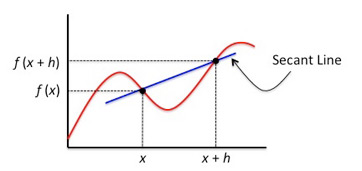Just to review, a function is a line or curve that has only one y value for every x value. It's like an input/output machine. For any number x that you plug into the function, you will get an output value for f (x).

In simple terms, the difference quotient helps us find the slope when we are working with a curve. In the case of a curve, we cannot use the traditional formula of: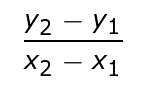which is why we must use the difference quotient formula.

In the formal definition of the difference quotient, you'll note that the slope we are calculating is for the secant line. A secant line is just any line that passes between two points on a curve. We label these two points as x and (x +h) on our x-axis. Because we are working with a function, these points are labeled as f (x) and f (x + h) on our y-axis, respectively.

Now that we understand the definition of the difference quotient, let's explore the formula.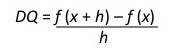## Finding f (x + h)

The first step necessary to finding the difference quotient is to find our f (x + h). When working with a function, all you have to do is plug (x + h) into your function wherever you see an x.

Let's look at the function: f (x) = 2x + 6.

To find our f (x + h), we need to plug in (x + h) into the function wherever we see an x.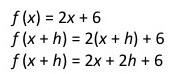Once we have our (x + h) plugged into the function, we must simplify our expression. In this case, we multiplied everything inside the parentheses by two to get:

F(x+h) = 2x + 2h + 6

Let's look at a more difficult function: f (x) = 3x^2 + 4.

Again, we must plug (x + h) into the function wherever we see an x.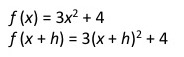Now, to simplify the expression, we must use the FOIL method to expand our (x + h)^2. Remember, FOIL stands for:

• Multiply the first numbers: 3(x+h)^2
• Multiply the outer numbers: 3(x+h) (x+h)
• Multiply the inner numbers: 3 (x^2 +xh+xh+h^2)
• Multiply the last numbers: 3 (x^2+2xh+h^2)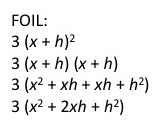We then plug our foiled expression back into the function to get: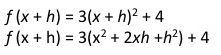To finish simplifying the expression, we multiply everything inside the parentheses by three.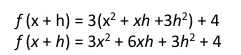Not too difficult so far, right?

## Finding the Difference Quotient

Now that we understand how to find f (x + h), we can plug our values into the difference quotient formula and simplify from there.

Let's use our earlier example of f (x) = 3x^2 + 4.

We can plug in the expression we found for f (x + h) and our expression for f (x) to get a difference quotient of: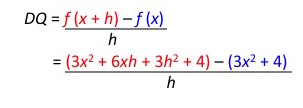It is extremely important to keep each segment of the difference quotient inside of it's own set of parentheses. This means that f (x + h) should be inside of it's own set of parentheses and f (x) should be inside of it's own set of parentheses.

## Step Simplifying & Solving

This step is where some students make mistakes when working with the difference quotient. It is important to take note of the subtraction sign between f (x + h) and f (x). This subtraction sign tells us to change the sign in everything inside of the parentheses to the right of it. This allows us to get rid of our parentheses.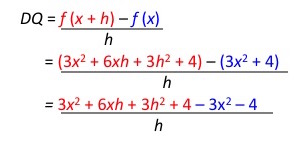To unlock this lesson you must be a Study.com Member.

### Register to view this lesson

Are you a student or a teacher?

### Unlock Your Education

#### See for yourself why 30 million people use Study.com

##### Become a Study.com member and start learning now.
Back
What teachers are saying about Study.com

### Earning College Credit

Did you know… We have over 160 college courses that prepare you to earn credit by exam that is accepted by over 1,500 colleges and universities. You can test out of the first two years of college and save thousands off your degree. Anyone can earn credit-by-exam regardless of age or education level.